# Volume Of Rectangular Prisms & Cubes

in hive-122481 •  17 days ago

Hi there. In this post, I go over the volume of rectangular prisms and cubes. It is assumed the reader is familiar with multiplication. One extension of this volume topic is introducing high school algebra (Grade 9 to 11) into the mix.

Math text/images rendered with QuickLaTeX.com.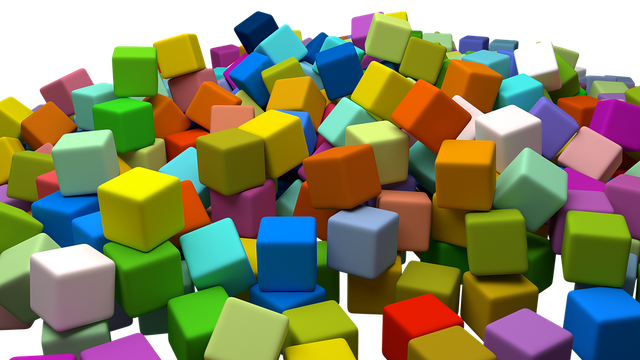Pixabay Image Source

## Topics

• Volume - The Amount Of Space In A 3D Shape
• Finding The Volume Of A Rectangular Prism
• Volume Of Cubes
• Extension - Combining Algebra With Volume

## Volume - The Amount Of Space In A 3D Shape

Volume refers to the amount of space something takes up in a three-dimensional object. Another simpler definition of volume is the amount of space inside a 3D object or container.

The units for volume are in cubic units.Duckduckgo Image Search Source

## Finding The Volume Of A Rectangular Prism

Given a rectangular prism, the volume inside it is quite simple. A rectangular prism volume is the length of the prism multiplied by its width and then multiplied by the height. In math formula notation it looks like this: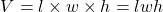where:

• l is the length of the prism
• w is the width of the prism
• h is the height of the prism

Another way of looking at volume is multiplying the area of the prism's base by the (vertical) height of the prism. The adjusted formula would like this: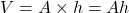where:

• A is the area of the prism base (length times width)
• h is the height of the prism

Rectangular Prism Practice Problems

Q1 A small box has a width of 5 centimetres, a height of 3 centimetres and a length of 8 centimetres. What is the volume of this box?

Q2 A big box has has a width of 40 centimetres, a height of 1 metre and a length of 50 centimetres. What is the volume of this box?

Q3 Repeat question one again but with all the dimensions doubled. How much larger is this box compared to the small box in question one?

## Volume Of Cubes

Just like how a square is a special case of a rectangle, a cube is a special case of a rectangular prism. The square has the length and width being of the same measure. Likewise, the cube has the length has the same measure as the cube's width and its height.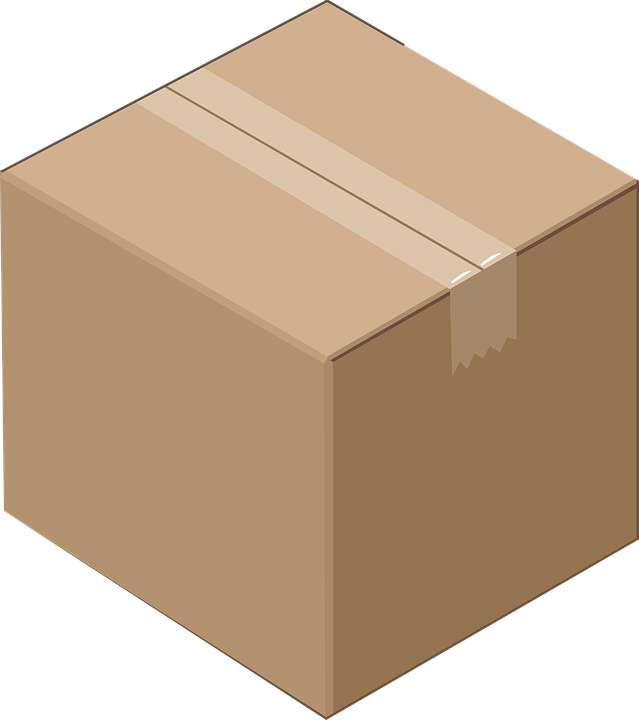Pixabay Image Source

For the cube, the formula is slightly different. The volume is the length multiplied by itself multiplied by itself. You can also substitute the length with the cube's width or height as the length is the same measure as the width and the height.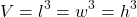Note that cube numbers are formed from whole numbers to the power of three. In other words, a positive whole number multiplied by itself twice gives a cube number. For example, the cube number of 2 is 8 as 2 x 2 x 2 = 8.

Cube Practice Problems

Q1 What is the volume of a cube with a side length of 10 metres?

Q2 Determine the volume of a cube with a height of half of a metre?

Q3 A cube's square base perimeter is 12 centimetres. What is the volume of this small cube?

## Extension - Combining Algebra With Volume

A harder version of the volume problems would be adding in some algebra. Instead of having everything with known numeric measurements, unknown measurements can be added.

Example One

A rectangular prism has a length of x + 7, a width length of x and a height of 8. What is the volume of this prism in cubic units? (It is assumed that x is a positive number.)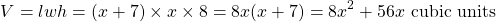Example Two

Given a rectangular prism volume of 800x and a base area of 40, what is the height of the prism?

From the volume formula we have: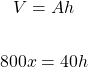Solving for x (through division) gives h = 20x.

Combining Algebra With Volume Practice Problems

Here are some practice problems. These questions are for high school math students (grades 10 to 11). The bonus one is more advanced.

Q1 What is the volume of a cube with a side length of 2x?

Q2 Determine the volume of the rectangular prism with a length of (x + 4), a width of x and a heigh of (x + 10).

Q3 A rectangular prism with a volume of 2(x^2 - 9) has a height of (x + 3). Find the area of the prism's base.

BONUS) A rectangular prism has a volume of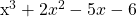. Assume that x is a positive integer, the height is greater than the length and the length is larger than the width. Determine the dimensions of this prism. (Hint: This would require the Factor Theorem from grade 12 mathematics / pre-calculus.)Pixabay Image Source

Answers To Rectangular Prism Practice Problems

Q1 A small box has a width of 5 centimetres, a height of 3 centimetres and a length of 8 centimetres. What is the volume of this box?

Q2 A big box has has a width of 40 centimetres, a height of 1 metre and a length of 50 centimetres. What is the volume of this box?

Q3 Repeat question one again but with all the dimensions doubled. How much larger is this box compared to the small box in question one?

Answer: Doubling the width, height and length gives 10 x 6 x 16 = 960 cubic centimetres. This new volume is 8 times larger than the small box volume.

Q1 What is the volume of a cube with a side length of 10 metres?

Q2 Determine the volume of a cube with a height of half of a metre?

Q3 A cube's square base perimeter is 12 centimetres. What is the volume of this small cube?

Answer: Given that the square base perimeter is 12 centimetres, dividing by 4 (for four sides) gives a side length of 3 centimetres. The volume of this small cube is 3 centimetres cubed which is 27 cubic centimetres.

Combining Algebra With Volume Practice Problems

Q1 What is the volume of a cube with a side length of 2x?

Answer: Raising 2x to the power of three gives 8x^3.

Q2 Determine the volume of the rectangular prism with a length of (x + 4), a width of x and a height of (x + 10).

Answer: Volume = x^3 + 14x^2 + 40x

Q3 A rectangular prism with a volume of 2(x^2 - 9) has a height of (x + 3). Find the area of the prism's base.

Answer: Factoring the difference of squares gives (x - 3)(x + 3). Dividing the volume by the height of (x + 3) gvies the prism base area of 2(x - 3).

BONUS) A rectangular prism has a volume of. Assume that x is a positive integer larger than 2, the height is greater than the length and the length is larger than the width. Determine the dimensions of this prism. (Hint: This would require the Factor Theorem from grade 12 mathematics / pre-calculus.)

Answer: Factored Product is (x + 1)(x - 2)(x + 3). Height is (x + 3), length is (x + 1) and width is (x - 2)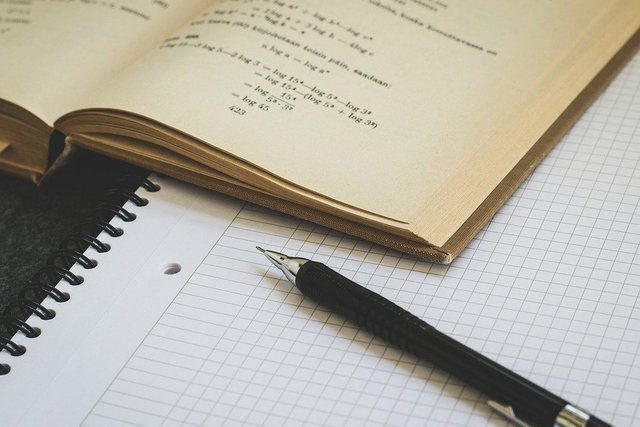Pixabay Image Source